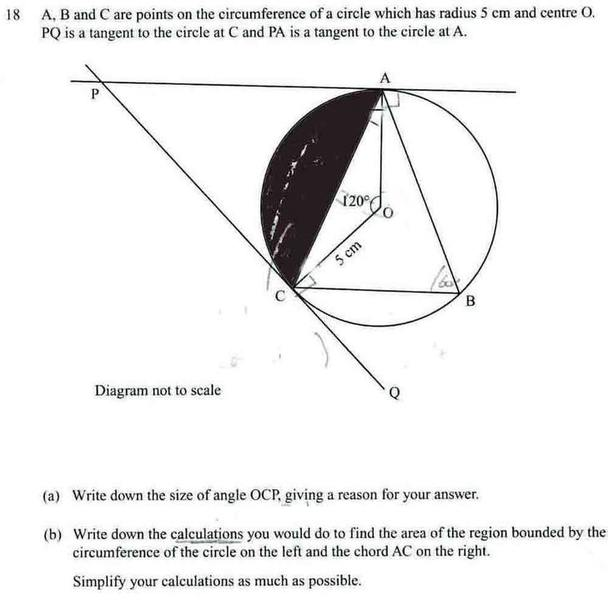# [GCSE] Simplifying segment

• thomas49th

#### thomas49th

Without using a calculator

This is part of a GCSE maths question from a non-calc paper

How does

$$\frac{25\pi}{3}-\frac{25}{2}sin120$$ become simplified?

What do you think? Do you know the value of sin120? (Hint: consider the graph of the sine function; sin120 is equal to the sine of an angle between 0 and 90)

sin120 is 0.866025...

but that's using a calculator

Ok, I'll rephrase my question, what's the exact value of sin120. Did you follow my hint and draw the sine graph? 120 degrees is 60 degrees to the left of 180. Now, since the graph is symmetric, the other angle x such that sinx=sin120 is ___ degrees to the right of zero, i.e. it is ___ degrees.

as you say the graph is symettric so sin60 = sin 120 = 0.866...

how does that help?

Ok, so sin120=sin60. Now, have you ever been taught the exact values for sin30, sin60, cos30, cos60? If you have, then you should use the exact value for sin60, if not, this is the way I was shown to do it.

Draw an equilateral triangle with sides with length 2-- each angle is 60 (since it's equilateral). Now, draw a line bisecting one of the angles, so you have two equal right angled triangles. Consider one of these; this will have sides of length 2, 1 (half of one of the equilateral triangle's sides) and √3 (by Pythagoras). Now, using the fact that sine=opposite/hypotenuse, you can obtain the exact value for sin60.

yeh we've been taught sin30 and cos 60, but I never recall the triangle method you know.

Here is the actual question (it's part b)I will test out your method now. However is it necessary? I looked in the answer booklet and it says

$$Area of sector AOC is \frac{120}{360} x \pi x h^{2}$$
$$Area of triangle AOC = \frac{1}{2} x 5 x 5 sin120$$
$$Area of segment = \frac{25\pi}{3}-\frac{25}{2}sin120 ---> 25(\frac{\pi}{3}-\frac{\sqrt{3}}{4})$$

Last edited:
It's not necessary if you know that sin60=√3/2. I was just showing a way to derive it, instead of just pulling the result out of a hat!

You can either memorise the common angles, or remember a way to derive them!

Thanks a lot :D, but in a exam would you use that method to answer part b?

The method you state in post number 7 is the correct way to answer the question. My method with the triangle is simply a way to work out sin60 (and the other angles) if you forget them. Personally, if I came across a question like that, then I would derive it using the triangle, purely becuase I'm pretty bad at memorising things! However, if you're going into an exam where you know that these questions will be asked, it may be better for you to memorise sine and cosine of 30, 60, 45. However, it's completely upto you.